•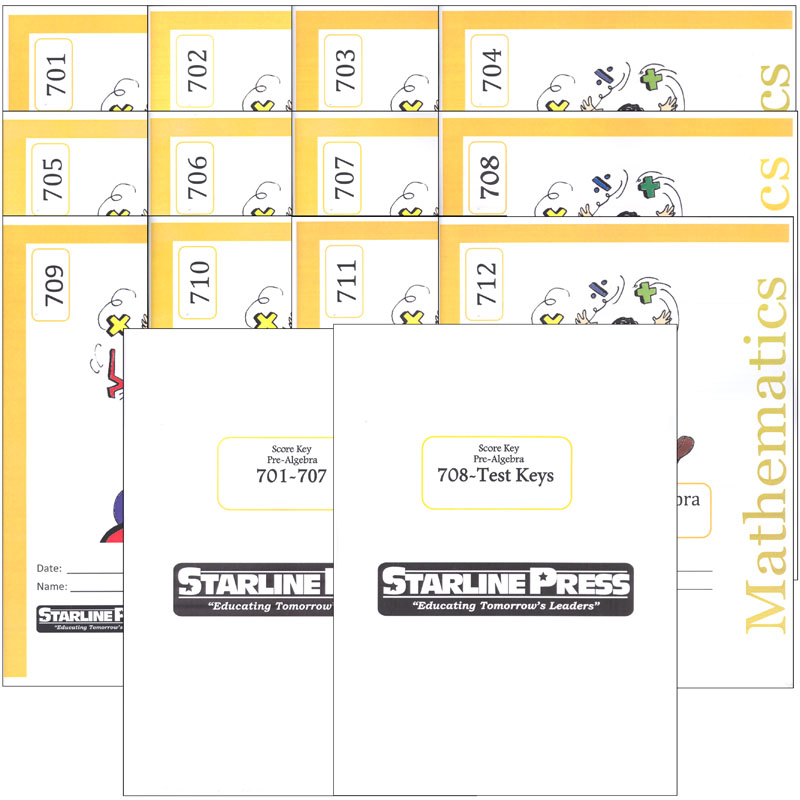•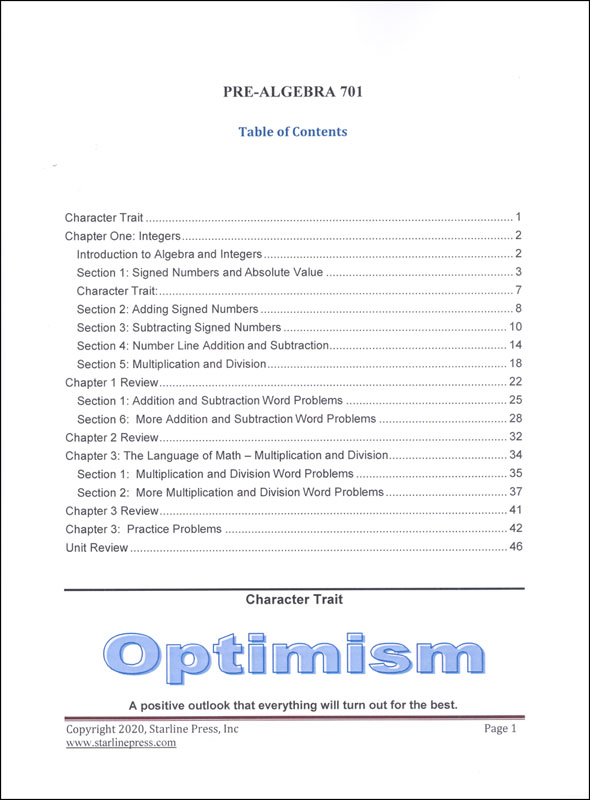•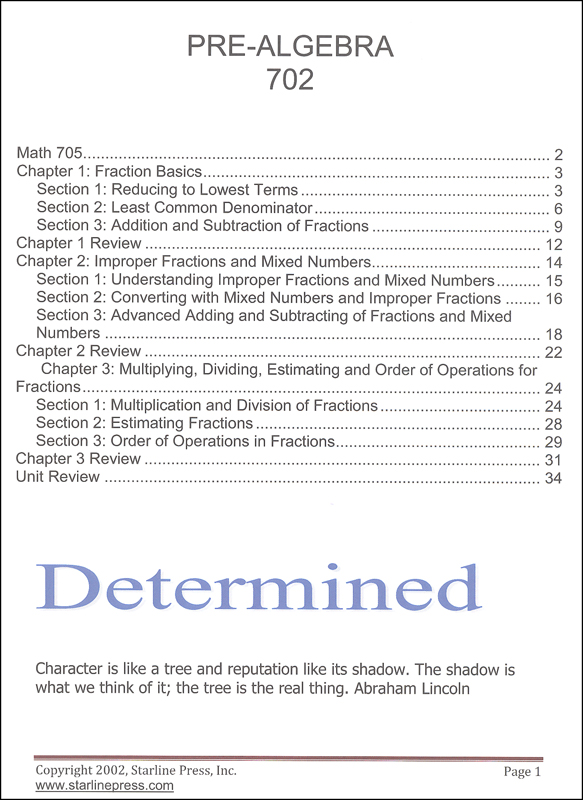•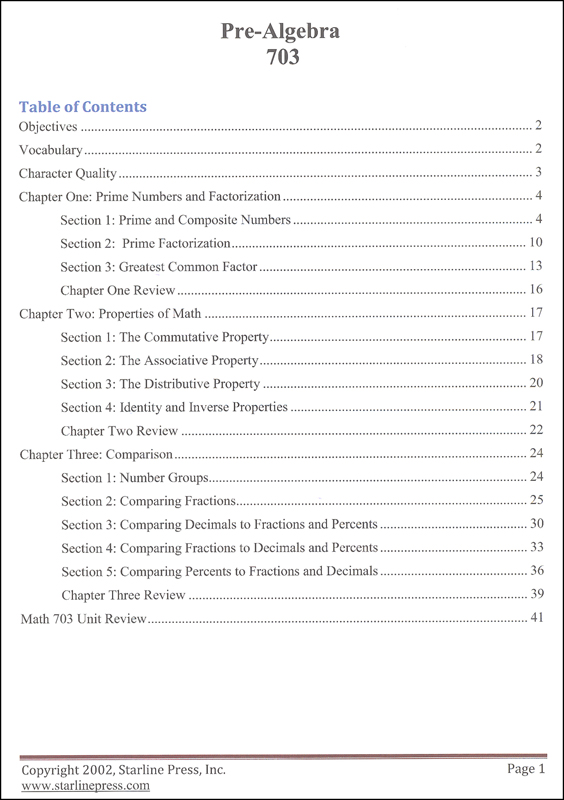•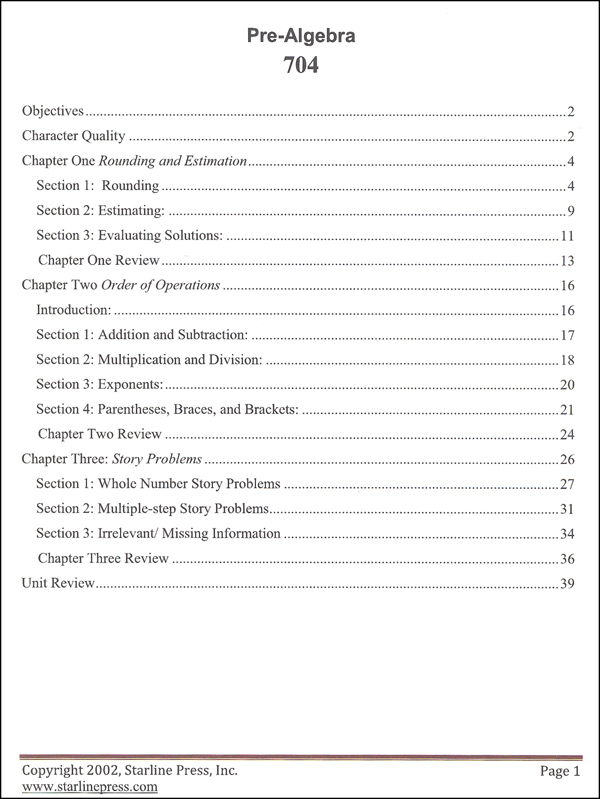•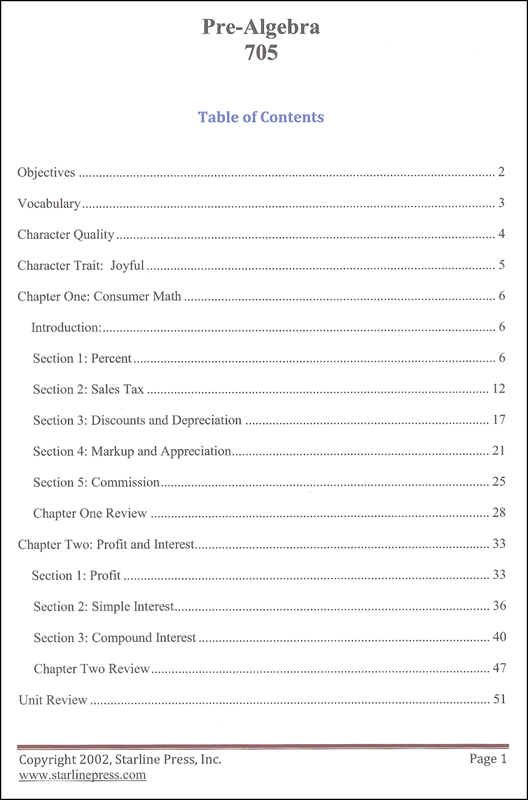•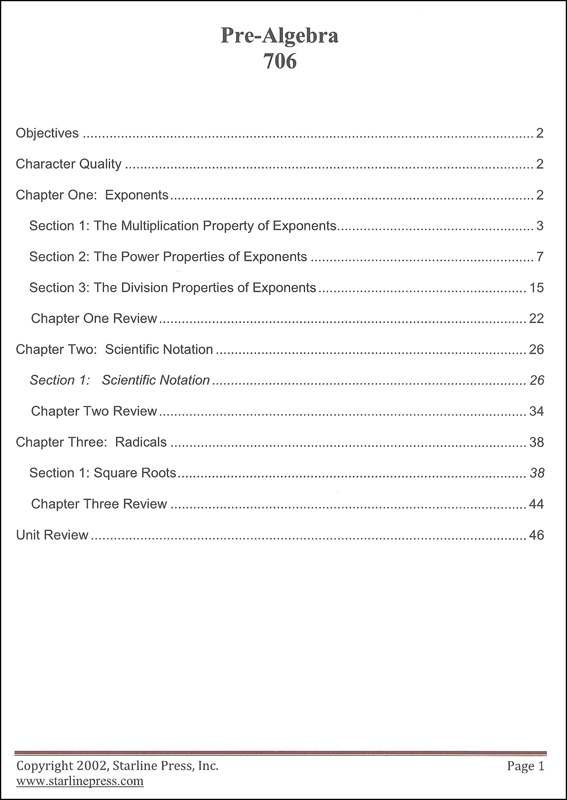•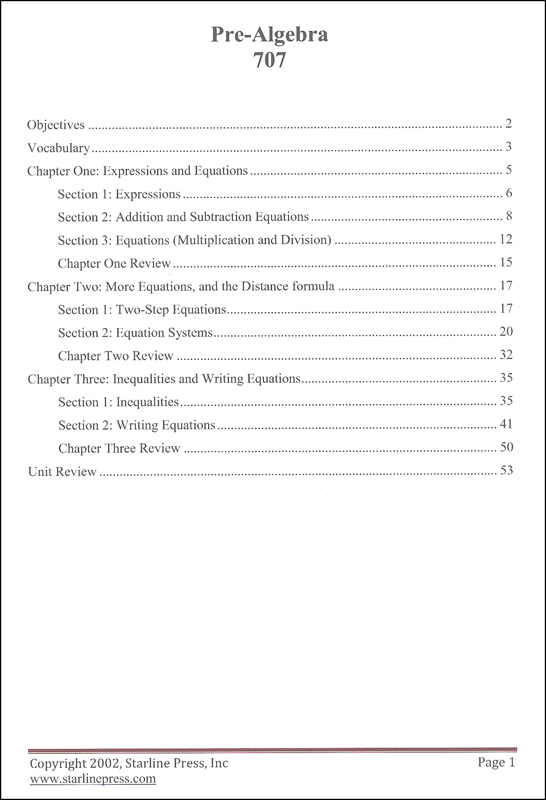•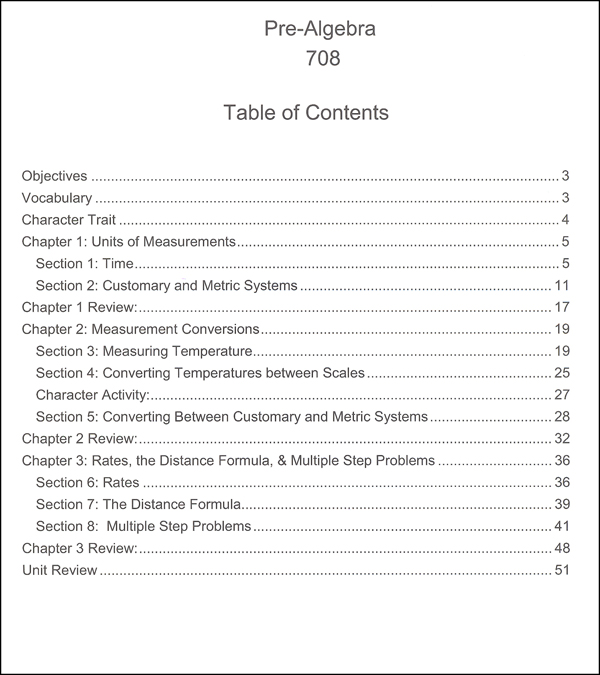•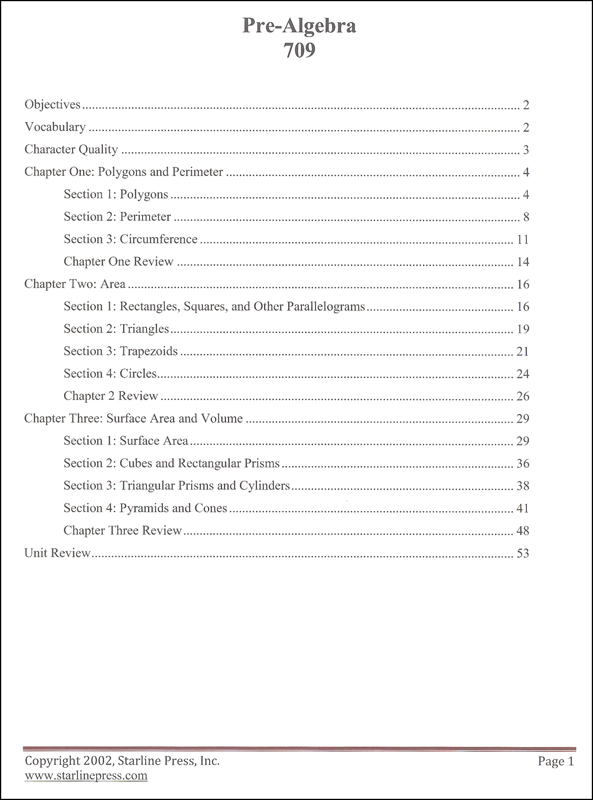•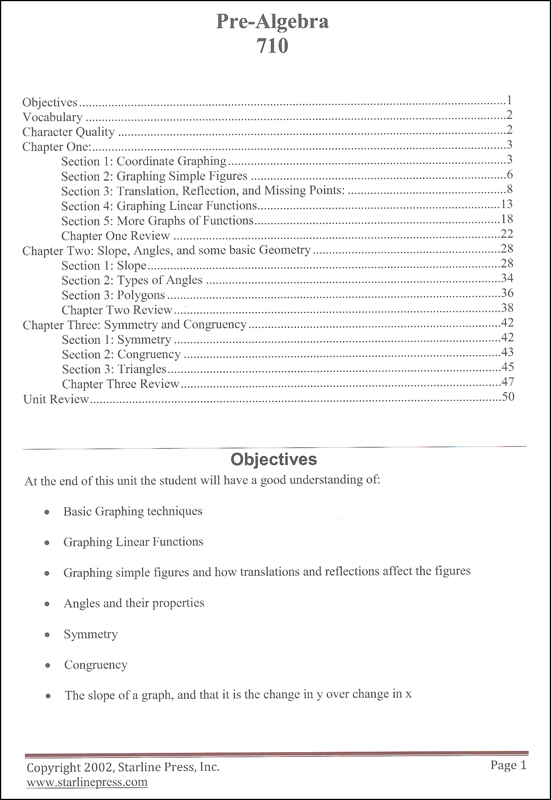•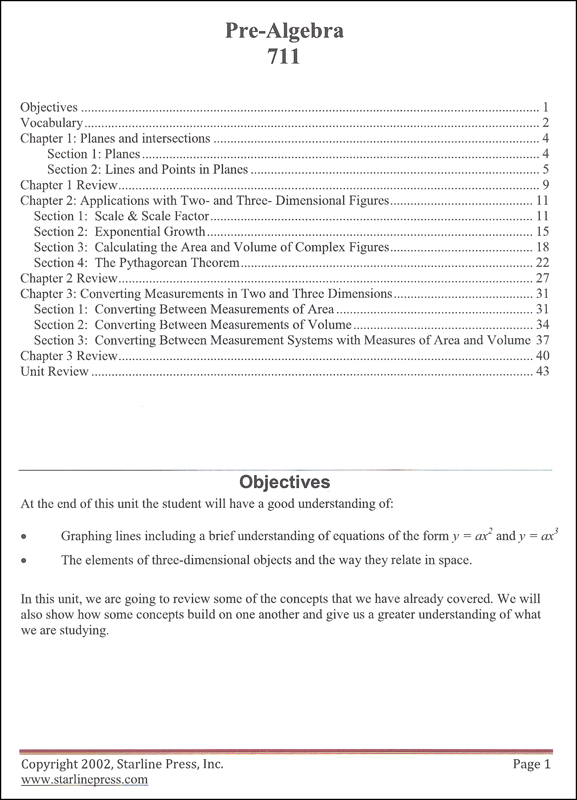•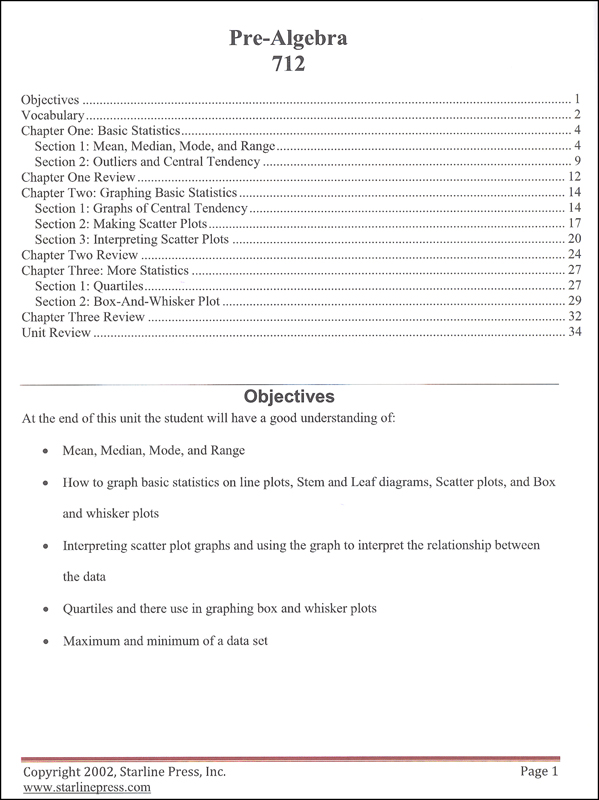•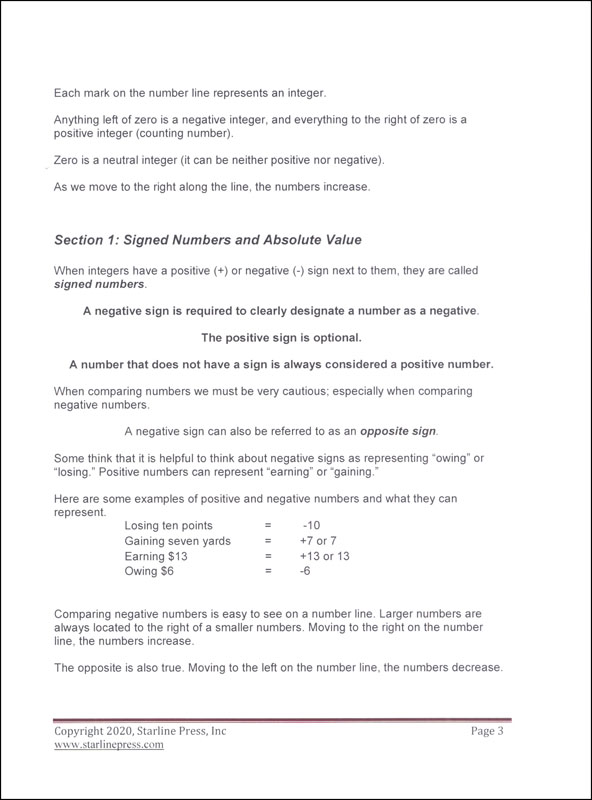•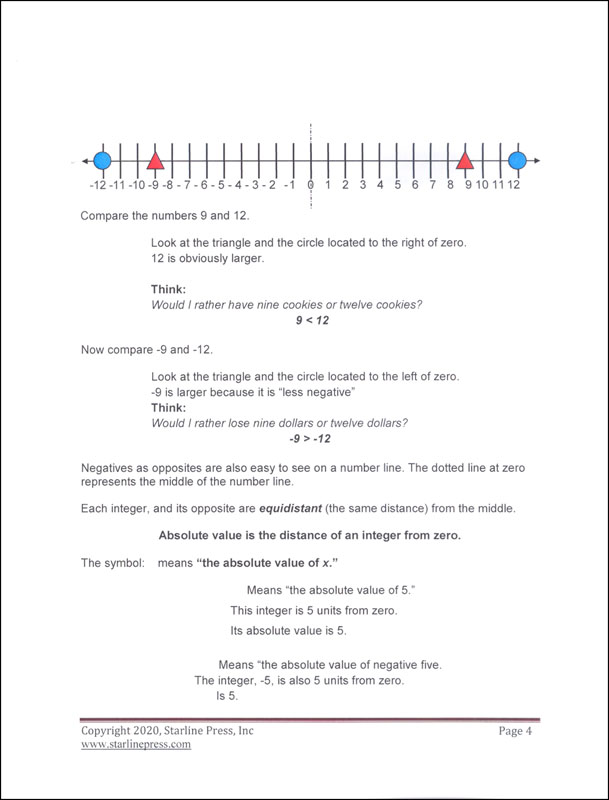•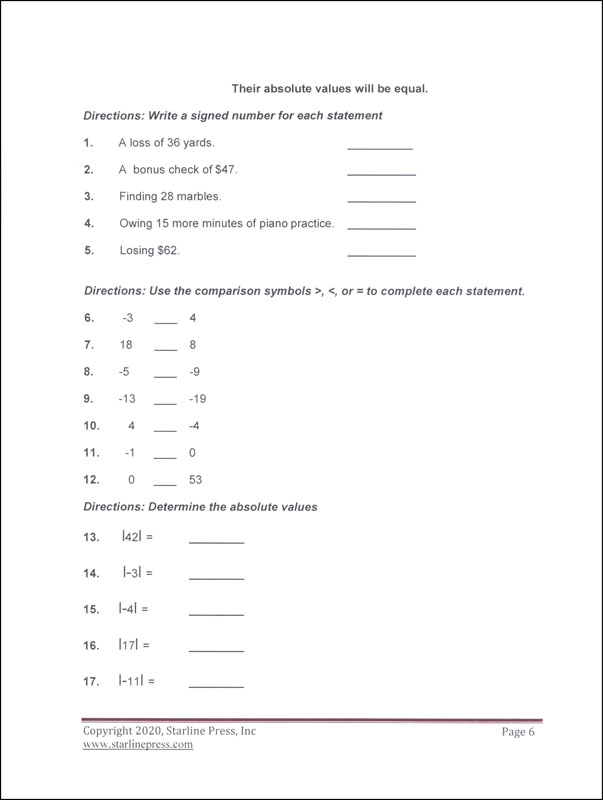•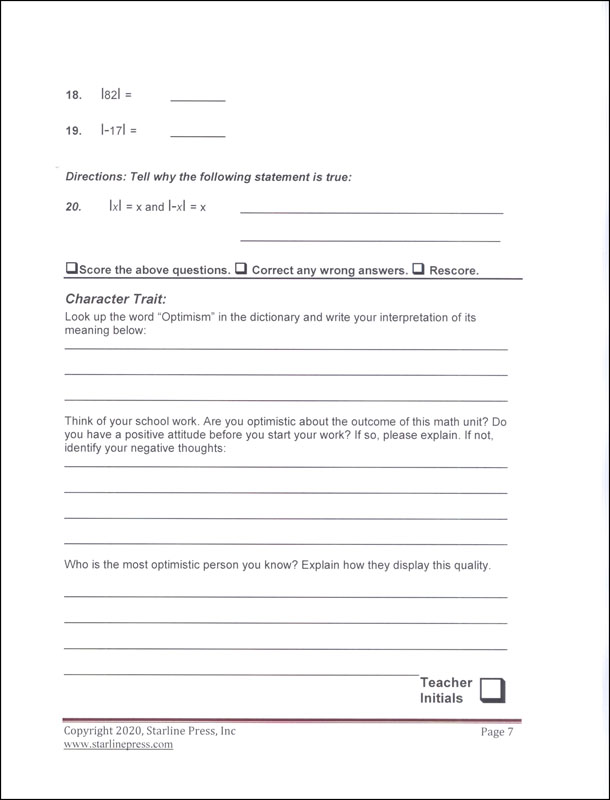•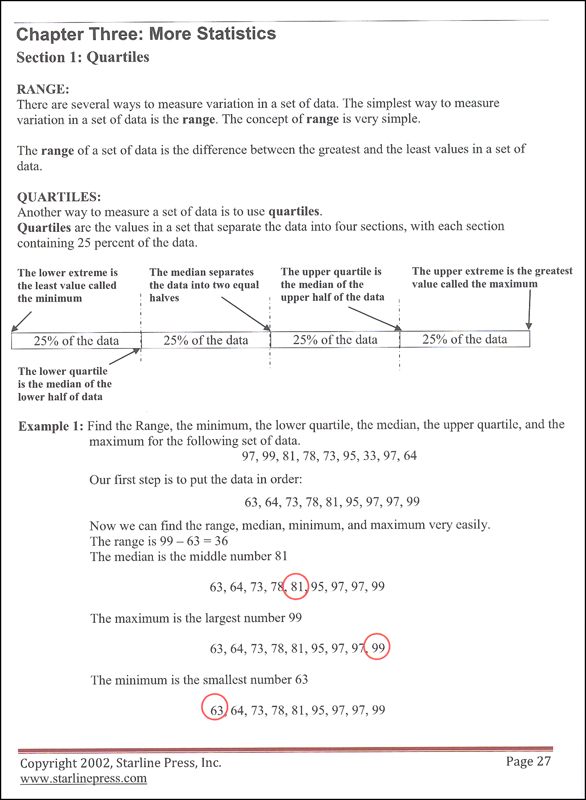# Pre-Algebra Set

# 051464

Our Price: \$200.95
Retail: \$218.71
Save: 8.12% (\$17.76)
In Stock.
Qty:
Qty:

 Item #: 051464 7

#### Product Description:

PRE-ALGEBRA: Seventh grade students study integers and word problems with addition/subtraction/multiplication/division (701); fractions (addition, subtraction, improper fractions, mixed numbers and the order of operations in fractions) (702); prime numbers, factorization, properties, comparing fractions/decimals/percents (703); rounding, estimation and order of operations (704); consumer math (sales tax, discounts, profit, interest and more) (705); exponents, scientific notation and radicals (706); expressions, equations and inequalities (707); units of measurement, unit conversion, rates and distance (708); polygons, perimeter, area, surface area and volume (709); graphing (coordinate, simple figures, linear functions), slope, angles, polygons, symmetry and congruency (710); planes, lines, points, exponential growth, unit conversions (711); statistics (basics, scatter plots, quartiles, box-and-whisker plots) (712). There are two keys: one for 701-707 and one for 708-712 and tests.

#### Publisher Description:

Pre-Algebra is an advanced course for seventh graders. This year-long course prepares students to enter the study of Algebra I (a high school credited course) as eighth graders. The focus of the course is building the foundation necessary for success in the study of algebra. Students will become familiar with the following: the properties of mathematics; the language of algebra; solving one step and two step equations; adding, subtracting, multiplying and dividing rational numbers and integers; solving inequalities; graphing equations and inequalities; proportion; percent; statistics and graphs; probability; applying algebra to geometry; measurement; introduction to trigonometry; and the study of polynomials.

Category Description for Starline Press Math:

Starline Press Math is a character-based, individualized and independent learning curriculum. Aligned to pre-Common Core California state standards (please see publisher website for CA state standards correlation), each grade level provides self- directed instruction with minimal teacher supervision. Grades 3-8 complete twelve units (booklets) per year, with a suggested 3 week completion time frame. Grades 9-10 complete 6 booklets per semester /12 per year. Each grade level includes vocabulary, fill in the blank questions, practice problems, chapter reviews, and unit tests. Consumable worktexts are colorfully illustrated soft covers, although newer printings are more like booklets and do not have the perfect binding and glossy covers. Available only in complete sets which include answer and test keys. ~ Deanne

Category Description for COMPREHENSIVE PROGRAMS - ALL GRADES:

Primary Subject
Mathematics
7
Format
Softcover Book Set
Brand Name
Starline Press
Weight
4.3187 (lbs.)
Dimensions
11.0" x 8.5" x 2.62"